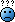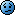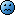###Author Topic: is anyone on this board competent in calculus ?  (Read 701 times)

#### coola

• Guest##### Re: is anyone on this board competent in calculus ?
« Reply #15 on: April 28, 2006, 09:38:03 AM »
thanks for that nibs and scarab..

Ask my Muslim brothers nibs and scarab (Kassem Fawzy), they'll take care of you.  The world has the Muslims to thank for Algebra (invented by Al-Jabeer), Arabic numerals, and the number 0.

yep i appreciate their help... and we have alot of cultures to thank for what the world is today...

#### E. J. Rizo

• The "Mac" Daddy
• Muthafuckin' Don!
•• Posts: 3601
• Karma: 193##### Re: is anyone on this board competent in calculus ?
« Reply #16 on: April 28, 2006, 09:38:59 AM »#### coola

• Guest##### Re: is anyone on this board competent in calculus ?
« Reply #17 on: April 28, 2006, 09:40:32 AM »
try this one !!

(3x^2) / ((y^2)+1) + y = 3x + 1

#### nibs

• Muthafuckin' Don!
••• Posts: 1191
• Karma: 1
• aco forever##### Re: is anyone on this board competent in calculus ?
« Reply #18 on: April 28, 2006, 10:01:35 AM »
try this one !!

(3x^2) / ((y^2)+1) + y = 3x + 1

3x^2((y^2)+1)^-1 + y = 3x + 1

6x((y^2)+1)^-1 - 3x^2((y^2)+1)^-2(2ydy/dx) + dy/dx = 3

6x((y^2)+1)^-1 - 6x^2(ydy/dx)((y^2)+1)^-2 + dy/dx = 3

6x((y^2)+1)^-1 - (6yx^2((y^2)+1)^-2 + 1)dy/dx = 3

6x((y^2)+1)^-1 - 3 = (6yx^2((y^2)+1)^-2 + 1)dy/dx

(6x / ((y^2) + 1) - 3) / ((6yx^2/((y^2)+1)^2) + 1)  =  dy/dx

it's been re-corrected.  dy/dx didn't have the '3' factor to factor out.

« Last Edit: April 28, 2006, 10:22:19 AM by nibs »
"a four letter word is going out to every single enemy" - kam

#### nibs

• Muthafuckin' Don!
••• Posts: 1191
• Karma: 1
• aco forever##### Re: is anyone on this board competent in calculus ?
« Reply #19 on: April 28, 2006, 10:22:33 AM »
!
"a four letter word is going out to every single enemy" - kam

#### K A I N

• Guest##### Re: is anyone on this board competent in calculus ?
« Reply #20 on: April 28, 2006, 10:23:23 AM »
Calculus II will kill you if you having problems with this...It's scary how I forgot all this shithttp://www.mathematicshelpcentral.com/lecture_notes/calculus_1.htm

btw fuck geometry
« Last Edit: April 28, 2006, 10:25:17 AM by Doggie KAIN »

#### coola

• Guest##### Re: is anyone on this board competent in calculus ?
« Reply #21 on: April 28, 2006, 10:40:20 AM »
yeah nibs, i was wondering wtf you changed the 3 to a 1 before... ima go eat some soup then put down on paper your workings... the solution is different in the text#### coola

• Guest##### Re: is anyone on this board competent in calculus ?
« Reply #22 on: April 28, 2006, 10:41:54 AM »
Calculus II will kill you if you having problems with this...It's scary how I forgot all this shithttp://www.mathematicshelpcentral.com/lecture_notes/calculus_1.htm

btw fuck geometry

yeah this is only my first year... its basic stuff and i'm strugglingmight need a tutor next year.. hot blonde will doprops for the web-site !

#### coola

• Guest##### Re: is anyone on this board competent in calculus ?
« Reply #23 on: April 28, 2006, 11:08:49 AM »
heres the solution... i'm hittin the sack, too much for one night..

[ 3((y^2) + 1)((y^2) - 2x + 1) ] / [ ((y^2) + 1)^2 - 6(x^2)y ]

fuck knows hot to get that#### nibs

• Muthafuckin' Don!
••• Posts: 1191
• Karma: 1
• aco forever##### Re: is anyone on this board competent in calculus ?
« Reply #24 on: April 28, 2006, 11:50:07 AM »
i factored wrong:

3x^2((y^2)+1)^-1 + y = 3x + 1

6x((y^2)+1)^-1 - 3x^2((y^2)+1)^-2(2ydy/dx) + dy/dx = 3

6x((y^2)+1)^-1 - 6x^2(ydy/dx)((y^2)+1)^-2 + dy/dx = 3

6x((y^2)+1)^-1 + (1 - 6yx^2((y^2)+1)^-2)dy/dx = 3

6x((y^2)+1)^-1 - 3 = (6yx^2((y^2)+1)^-2 - 1)dy/dx

(6x / ((y^2) + 1) - 3) / (6yx^2((y^2)+1)^-2 - 1)  =  dy/dx

then they simplified this further:

(6x / ((y^2) + 1) - 3) / (6yx^2((y^2)+1)^-2 - 1)  =  dy/dx

(6x((y^2)+1)^2 / ((y^2) + 1) - 3((y^2)+1)^2) / ( 6yx^2 - ((y^2)+1)^2)  =  dy/dx

(6x((y^2)+1) - 3((y^2)+1)^2) / ( 6yx^2 - ((y^2)+1)^2)  =  dy/dx

(6x((y^2)+1) - 3((y^2)+1)((y^2)+1)) / ( 6yx^2 - ((y^2)+1)^2)  =  dy/dx

[ 3((y^2)+1)(2x - (y^2) + 1) ] / [  6yx^2 - ((y^2)+1)^2 ]  =  dy/dx

factoring out the minus sign

[ 3((y^2)+1)((y^2) - 2x + 1) ] / [ ((y^2)+1)^2 - 6y(x^2) ]  =  dy/dx

"a four letter word is going out to every single enemy" - kam

#### Doggystylin

• Guest##### Re: is anyone on this board competent in calculus ?
« Reply #25 on: April 28, 2006, 12:33:47 PM »
thanks for that nibs and scarab..

Ask my Muslim brothers nibs and scarab (Kassem Fawzy), they'll take care of you.  The world has the Muslims to thank for Algebra (invented by Al-Jabeer), Arabic numerals, and the number 0.

well fuck him then cause i hate algebra

#### Shallow

• Muthafuckin' Don!
••• Posts: 7283
• Karma: 215
• I never had a digital pic of myself before##### Re: is anyone on this board competent in calculus ?
« Reply #26 on: April 28, 2006, 07:33:54 PM »
thanks for that nibs and scarab..

Ask my Muslim brothers nibs and scarab (Kassem Fawzy), they'll take care of you.  The world has the Muslims to thank for Algebra (invented by Al-Jabeer), Arabic numerals, and the number 0.

well fuck him then cause i hate algebra

He was just joking around. "Al-Jabeer", "Al-ge-bra". While the name algebra is devired from the arabic word "al-ğabr" or "al jebr", which I'm told stands for "reunion of broken parts", the idea of agebra as we know it goes as far back as the Babylonian times at least 4000 years ago. 2400 years before anyone was calling themselves a Muslim. Now Calculus as we know it today came about from Newton and his colleagues in Europe about 300 years ago.

#### Sikotic

• Muthafuckin' Don!
••• Posts: 28717
• Thanked: 4 times
• Karma: 3137
• PussyCunt##### Re: is anyone on this board competent in calculus ?
« Reply #27 on: April 29, 2006, 12:10:00 AM »
I just failed my calculus test today.THA SAUCE HOUSE

#### herpes

• Guest##### Re: is anyone on this board competent in calculus ?
« Reply #28 on: April 29, 2006, 05:47:43 AM »
I can count to 12